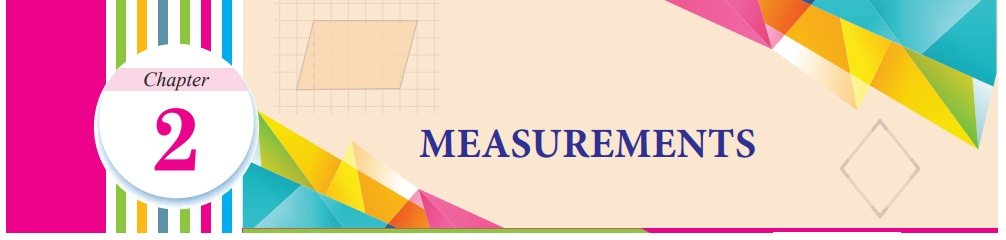Home | | Maths 7th Std | Measurements

# Measurements

Learning Objectives ● To recall the concepts of perimeter and area of square, rectangle, right angled triangle and combined shapes. ● To understand and find the area and perimeter of parallelogram, rhombus and trapezium.

Chapter 2

MEASUREMENTSLearning Objectives

● To recall the concepts of perimeter and area of square, rectangle, right angled triangle and combined shapes.

● To understand and find the area and perimeter of parallelogram, rhombus and trapezium.

Recap

In ancient days people used non-standardised measures such as cubit, king’s foot, king’s arm and yard etc. Later, people realised the need for standardised units and International System of Units which were introduced in the year 1971.The SI Units of various measures are shown in the table

In Class VI, we studied about area and perimeter of rectangle, square and right angled triangle.

Perimeter is the distance around and Area is the region occupied by the closed shape.

Try these :

Find the missing values for the following:Hint:

The perimeter of a rectangle = 2 × (l + b) units.

Area of a rectangle = l × b square units.

(l and b are length and breadth of a rectangle)Hint:

Perimeter of a rectangle = 1 × a units

Area of a square = a × a square units.

('a' is the side of the square)Hint:

Area of the right angled triangle = 1/2 (b × h) square units

(‘b’ is the base and ‘h’ is the height of the triangle)

Introduction

We have studied area of four sided shapes such as square and rectangle. Do you think that all the four sided shapes will happen to be square or rectangle? Think!

Let us learn about some more four sided shapes that we see around us through the conversation that follows.

Observe the shapes found in the figure

Teacher : Can you tell me the name of the shapes that you see in the figure?

Student : Yes teacher, there is a triangle, square and rectangle

Teacher : What is the shape, numbered 4 in the figure?

Student : The shape looks like a rectangle because the opposite sides are equal and parallel but the adjacent sides do not make right angle. Can we call such shapes as rectangles?

Teacher : Even though the opposite sides are equal and parallel, the adjacent sides do not make right angles instead they make acute and obtuse angles. This shape has a special name called parallelogram.Student : Teacher, can you tell me about shape, numbered 5?

Teacher : Try to tell the properties that you know, then I will help you.

Student : Shape 5 has all the sides equal and looks like a square but the adjacent sides do not make right angles. Is there any special name for such shapes.

Teacher : Yes, it has a special name called rhombus. What about the shape, numbered 6?

Student : Shape 6 does not have any of the properties of square and rectangle. But one pair of opposite sides are parallel. Does this also have a different name?

Teacher : Yes, it is called trapezium.

Now, let us learn about all the three new shapes namely parallelogram, rhombus and trapezium.

Tags : Term 1 Chapter 2 | 7th Maths , 7th Maths : Term 1 Unit 2 : Measurements
Study Material, Lecturing Notes, Assignment, Reference, Wiki description explanation, brief detail
7th Maths : Term 1 Unit 2 : Measurements : Measurements | Term 1 Chapter 2 | 7th Maths